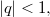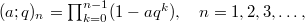﻿

### On some continued fraction expansions for the ratios of the function

#### Abstract

In his lost notebook, Ramanujan has defined the function$\rho(a,~b)$ by \begin{equation*} \rho(a ,~b) := \left(1 +\frac{1}{b}\right)\sum_{n=0}^{\infty} \frac{(-1)^{n}q^{n(n+1)/2} {a}^{n}{b}^{-n}}{(-aq)_{n}}, \end{equation*} where$|q|< 1,$ and$(a; q)_n = \prod_{k = 0}^{n-1}(1-aq^k),~~~n=1,2,3,\dots,$ and has given a beautiful reciprocity theorem involving$\rho(a,~b)$. In this paper we obtain some continued fraction expansions for the ratios of$\rho(a,~b)$ with some of its contiguous functions. We also obtain some interesting special cases of our continued fraction expansions which are analogous to the continued fraction identities stated by Ramanujan. \end

DOI Code: 10.1285/i15900932v33n2p35

Keywords: Basic hypergeometric series; q-continued fractions

Full Text: PDF